# How to Convert Military Time to Standard Time in Excel

This post will guide you how to convert military time to standard time with a formula in Excel. How do I convert from military time 17:29:30 to regular time 5:29:30PM in Excel.  How do I Convert Standard time to military time with a formula in Excel.

## Convert Military Time to Standard Time

Assuming that you have a list of data in range B1:B4, in which contain military time. And you wish to convert those times to standard time. How to do it. You can a formula based on the TIMEVALUE function, the LEFT function, the MID function and the RIGHT function. The formula is similar to the below:

`=TIMEVALUE(LEFT(B1,2)&":"&MID(B1,3,2)&":"&RIGHT(B1,2))`

#1 You need to type this formula into a blank cell (at this example, we select Cell C1) and press Enter key, then copy this formula from cell C1 to range C2:C4.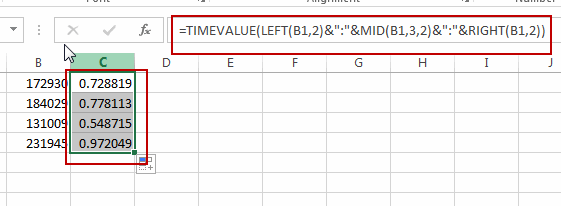#2 Then you need to select range C1:C4, and right click on it, select Format Cells from the popup menu list. the Format Cells dialog will open.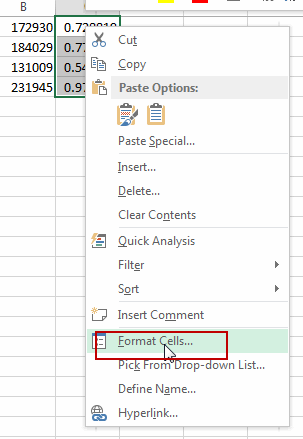#3 Switch to Number tab in the Format Cells dialog box, and click Time from the Category list box, then select the standard time type in the Type list box. Click Ok button.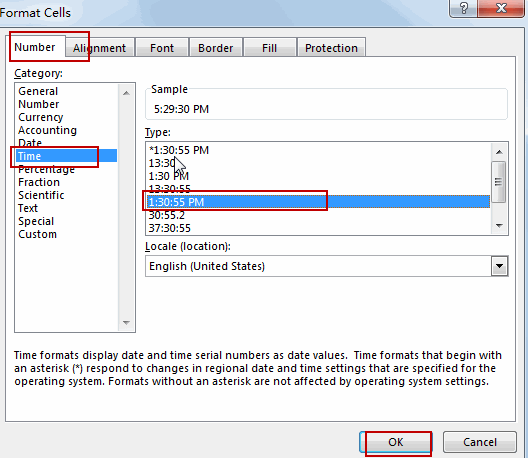#4 Let’s see the last result: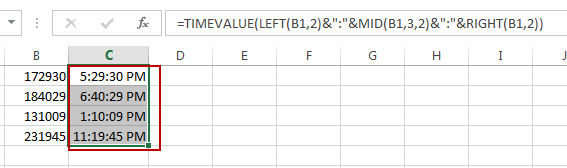## Convert Standard Time to Military Time

If you want to convert Standard time to Military time, you can use another formula based on the TEXT function. Like this:

`=TEXT(C1,"HHMMSS")`

Type this formula into cell D1, press Enter key on your keyboard. Drag the AutoFill Handle over to other cells to apply this formula.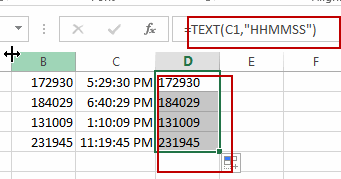### Related Functions

• Excel TIMEVALUE Function
The Excel TIMEVALUE function returns the decimal number of the time represented by a text string. so it will convert a time represented by a text string into an Excel time value.The syntax of the TIMEVALUE function is as below:=TIMEVALUE (time_text)…
• Excel MID function
The Excel MID function returns a substring from a text string at the position that you specify.The syntax of the MID function is as below:= MID (text, start_num, num_chars)…
• Excel LEFT function
The Excel LEFT function returns a substring (a specified number of the characters) from a text string, starting from the leftmost character.The LEFT function is a build-in function in Microsoft Excel and it is categorized as a Text Function.The syntax of the LEFT function is as below:= LEFT(text,[num_chars])…
• Excel RIGHT function
The Excel RIGHT function returns a substring (a specified number of the characters) from a text string, starting from the rightmost character.The syntax of the RIGHT function is as below:= RIGHT (text,[num_chars])…
• Excel Text function
The Excel TEXT function converts a numeric value into text string with a specified format. The TEXT function is a build-in function in Microsoft Excel and it is categorized as a Text Function. The syntax of the TEXT function is as below: = TEXT (value, Format code)…
Related Posts
How to Convert Text to Time in Excel

This post will guide you how to convert time string to time in Excel. Assuming that you have a list of text string in your worksheet, and you wish to convert these time string to standard time format, how to ...

How to Combine Text and Date into one Cell in Excel

This post will guide you how to combine text and dates in the same cell in Excel. How do I combine text and date values into one Cell in Excel 2013/2016. Combine Text and Date into One Cell Assuming that ...

How to Highlight Cell or Row If Date Is In Current Day/Week/Month in Excel

This post will guide you how to highlight cell if date is the current day or is in the current week or month in Excel. How do I highlight row if date is in current week or month with conditional ...

How to Delete or Remove Year from a Date in Excel

This post will guide you how to delete or remove year from a given standard date in Excel. How do I remove the year from a date with a formula in Excel. Remove Year from a Date Assuming that you ...

How to Convert Date to YYYY-MM-DD format in Excel

This post will guide you how to convert the current date to a specified date format in Excel. How do I convert date to YYYY-MM-DD format with Format Cells Feature in Excel. How to convert date format to a specific ...

How to Concatenate Cells and keeping Date Format in Excel

This post will guide you how to concatenate cells and keeping data format in Excel. How do I concatenate cells and retain the certain Date format with a formula in Excel 2010/2013/2016. Concatenate Cells and Keeping Date Format Assuming that ...

How to Display Negative Time Value in Excel

This post will guide you how to display negative time value in Excel. How do I change the way negative times are displayed in Excel. How to show negative dates or times with a formula in Excel. Display Negative Time ...

Extract Month and Year from Date in Excel

This post will guide you how to extract month and year from a date in excel. How do I get month and year from date cells  with a formula in Excel. How to convert date to month and year in ...

Convert Date to Text

This post will guide you how to convert dates stored as text format in Excel. How do I convert the date as date format to text format in excel. You can use a variety of formulas to convert the dates ...

Changing Date Format

This post will guide you how to convert dates between Australian and US American formats in Excel. How to change the data format from your local locale to other locale, such as: US or South Africa. As the different country ...

Sidebar